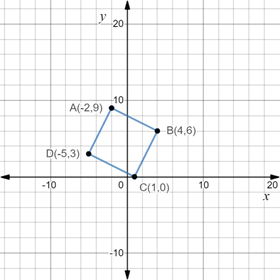# To verify: The given points are the vertices of a square.### Precalculus: Mathematics for Calcu...

6th Edition
Stewart + 5 others
Publisher: Cengage Learning
ISBN: 9780840068071### Precalculus: Mathematics for Calcu...

6th Edition
Stewart + 5 others
Publisher: Cengage Learning
ISBN: 9780840068071

#### Solutions

Chapter 1.8, Problem 41E
To determine

## To verify: The given points are the vertices of a square.

Expert Solution

### Explanation of Solution

Given information:

The four points A(2,9),B(4,6),C(1,0) and D(5,3) .

Formula used:

Distance formula between two points X(a1,b1) and Y(a2,b2) is calculated as,

d(XY)=(a2a1)2+(b2b1)2

A rhombus is a special kind of quadrilateral with all sides equal and square is a rhombus with equal lengths of diagonals.

Proof:

Consider the given four points A(2,9),B(4,6),C(1,0) and D(5,3) .

Plot the given points on coordinate plane and join the vertices to form the quadrilateral ABCD as,From the above figure, calculate the distance between all the four points A, B, C and D using distance formula to measure the length of sides.

Recall that the distance formula between two points X(a1,b1) and Y(a2,b2) is calculated as,

d(XY)=(a2a1)2+(b2b1)2

So, lengths of sides AB, BC, CA and DA will be calculated as,

d(AB)=(4(2))2+(69)2=(4+2)2+(3)2=36+9=45

d(BC)=(14)2+(06)2=(3)2+(6)2=9+36=45

d(CD)=(51)2+(30)2=(6)2+(3)2=36+9=45

d(DA)=(5(2))2+(93)2=(5+2)2+(6)2=9+36=45

Recall that a rhombus is a special kind of quadrilateral with all sides equal and square is a rhombus with equal lengths of diagonals.

Since, all the sides are equal, so, the given points are of rhombus.

Now, calculate the lengths of diagonals AC and BD,

d(AC)=(1(2))2+(09)2=(1+2)2+(9)2=9+81=90

d(BD)=(54)2+(36)2=(9)2+(3)2=81+9=90

Since, the diagonals are also of equal lengths, so, this rhombus is a square.

Since, the quadrilateral formed from the given points has equal sides and equal diagonals, thus it is proved that the given points are the vertices of a square.

### Have a homework question?

Subscribe to bartleby learn! Ask subject matter experts 30 homework questions each month. Plus, you’ll have access to millions of step-by-step textbook answers!